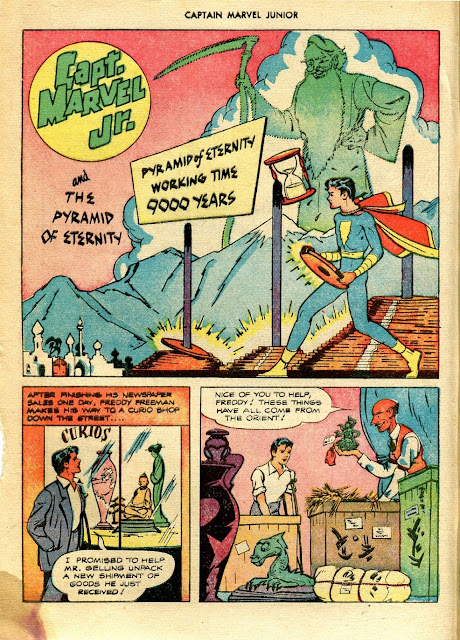## Monday, December 30, 2013

### A Golden Age Comic Book introduction to Recursion -- The Pyramid of Eternity

From 1947. this Captain Marvel Jr. story has a surprisingly mathematical bent.And a little later...

and a bit later still...

"The Pyramid of Eternity" is better known as the Towers of Hanoi and it's often used as example of recursion, particularly in programming courses. Here's the Wikipedia explanation:
A key to solving this puzzle is to recognize that it can be solved by breaking the problem down into a collection of smaller problems and further breaking those problems down into even smaller problems until a solution is reached. For example:
• label the pegs A, B, C — these labels may move at different steps
• let n be the total number of discs
• number the discs from 1 (smallest, topmost) to n (largest, bottommost)
To move n discs from peg A to peg C:
1. move n−1 discs from A to B. This leaves disc n alone on peg A
1. move disc n from A to C
1. move n−1 discs from B to C so they sit on disc n
The above is a recursive algorithm: to carry out steps 1 and 3, apply the same algorithm again for n−1. The entire procedure is a finite number of steps, since at some point the algorithm will be required for n = 1. This step, moving a single disc from peg A to peg B, is trivial.